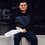# Cauchy-Schwarz Inequality: Equality Case

In this note, we will show the necessary conditions for two sequences of reals, $a_k$ and $b_k$ such that equality is reached by the Cauchy-Schwarz Inequality. I believe this fact is already presented in the Cauchy-Schwarz Brilliant Wiki, but I don't believe it was shown in this manner. First, we begin with a statement of the inequality:

$\left(\sum_{k=1}^{n} a_kb_k\right)^2 \leq \left(\sum_{k=1}^{n} a_k^{2}\right)\left(\sum_{k=1}^{n} b_k^{2}\right)$

Now, subtracting to yield a non-negative result,

$\left(\sum_{k=1}^{n} a_k^{2}\right)\left(\sum_{k=1}^{n} b_k^{2}\right) - \left(\sum_{k=1}^{n} a_kb_k\right)^2 \geq 0$

This expression can be expanded and then neatly, intuitively compacted into summation notation. Also, we'll be leaving behind the inequality sign in exchange for an equal sign, since we're focusing on the equality case.

$(a_1^2+a_2^2+...+a_n^2)(b_1^2+b_2^2+...+b_n^2) - (a_1b_1 + a_2b_2 + ... + a_nb_n)^2 = 0$

$\left(\sum_{i=1}^{n}\sum_{j=1}^{n} a_i^{2}b_j^{2}\right) - \left(\sum_{i=1}^{n}\sum_{j=1}^{n} a_ib_ia_jb_j \right) = 0$

$\frac{1}{2}\left(\sum_{i=1}^{n}\sum_{j=1}^{n} a_i^{2}b_j^{2} + a_j^2b_i^2\right) - \left(\sum_{i=1}^{n}\sum_{j=1}^{n} a_ib_ia_jb_j \right) = 0$

Now, combining our double sums:

$\frac{1}{2}\left(\sum_{i=1}^{n}\sum_{j=1}^{n} a_i^{2}b_j^{2} -2a_ib_ja_jb_i + a_j^2b_i^2\right) = \frac{1}{2}\left(\sum_{i=1}^{n}\sum_{j=1}^{n} (a_ib_j - a_jb_i)^2 \right) = 0$

If $(b_1, b_2, ... , b_n) \neq 0$, then $b_k \neq 0$ for some $k$. For equality to occur, the above expression must equal $0$, and so each term of the double sum must equal $0$. Considering only the positive terms $b_k$, it follows that equality holds with the Cauchy-Schwarz Inequality if and only if $a_kb_i = a_ib_k$ for all $i$ such that $1 \leq i \leq n$ and for some value of $k$.

Dividing both sides of this relation by $b_i$ and $b_k$ and setting $\gamma = \frac{a_k}{b_k}$, we reach the fact that $\frac{a_i}{b_i} = \gamma$. In other words, our sequences $a_k$ and $b_k$ must be $\textit{proportional}$ for the equality case of the Cauchy-Schwarz Inequality.Note by Ryan Tamburrino
6 years, 5 months ago

This discussion board is a place to discuss our Daily Challenges and the math and science related to those challenges. Explanations are more than just a solution — they should explain the steps and thinking strategies that you used to obtain the solution. Comments should further the discussion of math and science.

When posting on Brilliant:

• Use the emojis to react to an explanation, whether you're congratulating a job well done , or just really confused .
• Ask specific questions about the challenge or the steps in somebody's explanation. Well-posed questions can add a lot to the discussion, but posting "I don't understand!" doesn't help anyone.
• Try to contribute something new to the discussion, whether it is an extension, generalization or other idea related to the challenge.

MarkdownAppears as
*italics* or _italics_ italics
**bold** or __bold__ bold
- bulleted- list
• bulleted
• list
1. numbered2. list
1. numbered
2. list
Note: you must add a full line of space before and after lists for them to show up correctly
paragraph 1paragraph 2

paragraph 1

paragraph 2

[example link](https://brilliant.org)example link
> This is a quote
This is a quote
    # I indented these lines
# 4 spaces, and now they show
# up as a code block.

print "hello world"
# I indented these lines
# 4 spaces, and now they show
# up as a code block.

print "hello world"
MathAppears as
Remember to wrap math in $$ ... $$ or $ ... $ to ensure proper formatting.
2 \times 3 $2 \times 3$
2^{34} $2^{34}$
a_{i-1} $a_{i-1}$
\frac{2}{3} $\frac{2}{3}$
\sqrt{2} $\sqrt{2}$
\sum_{i=1}^3 $\sum_{i=1}^3$
\sin \theta $\sin \theta$
\boxed{123} $\boxed{123}$

Sort by:

interesting! I love the proof, especially the usage of summations. But I think the last portion of the argument is not valid.

Dividing both sides of this relation by bi and bk.

We only assumed bk to be non-zero. bi could be zero, making division by b_i wrong. Is that not true?

- 2 years, 5 months ago

Considering only the positive terms b_k

Did you mean non-zero? b_k could be negative, no?

- 2 years, 5 months ago